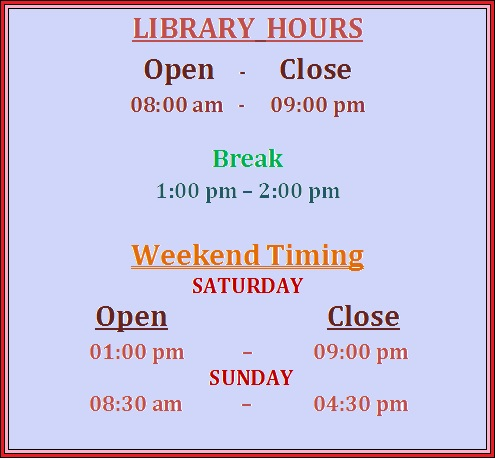# Introduction to Stochastic Processes: Home

Understand the notion of a Markov chain, and how simple ideas of conditional probability and matrices can be used to give a thorough and effective account of discrete-time Markov chains.

## Objectives

Understand the notion of a Markov chain, and how simple ideas of conditional probability and matrices can be used to give a thorough and effective account of discrete-time Markov chains, understand notions of long-time behavior including transience, recurrence, and equilibrium and  be able to apply these ideas to answer basic questions in several applied situations including genetics, branching processes and random walks. Be able to formulate continuous-time Markov chain models for applied problems. Be able to solve for the transition probabilities for Markov chains on a finite state space.

## Level`Graduate`

## Welcome

Welcome to the Library Guide for Introduction to stochastic processes. It will help you to locate key resources regarding to your subject.

## Library Timing